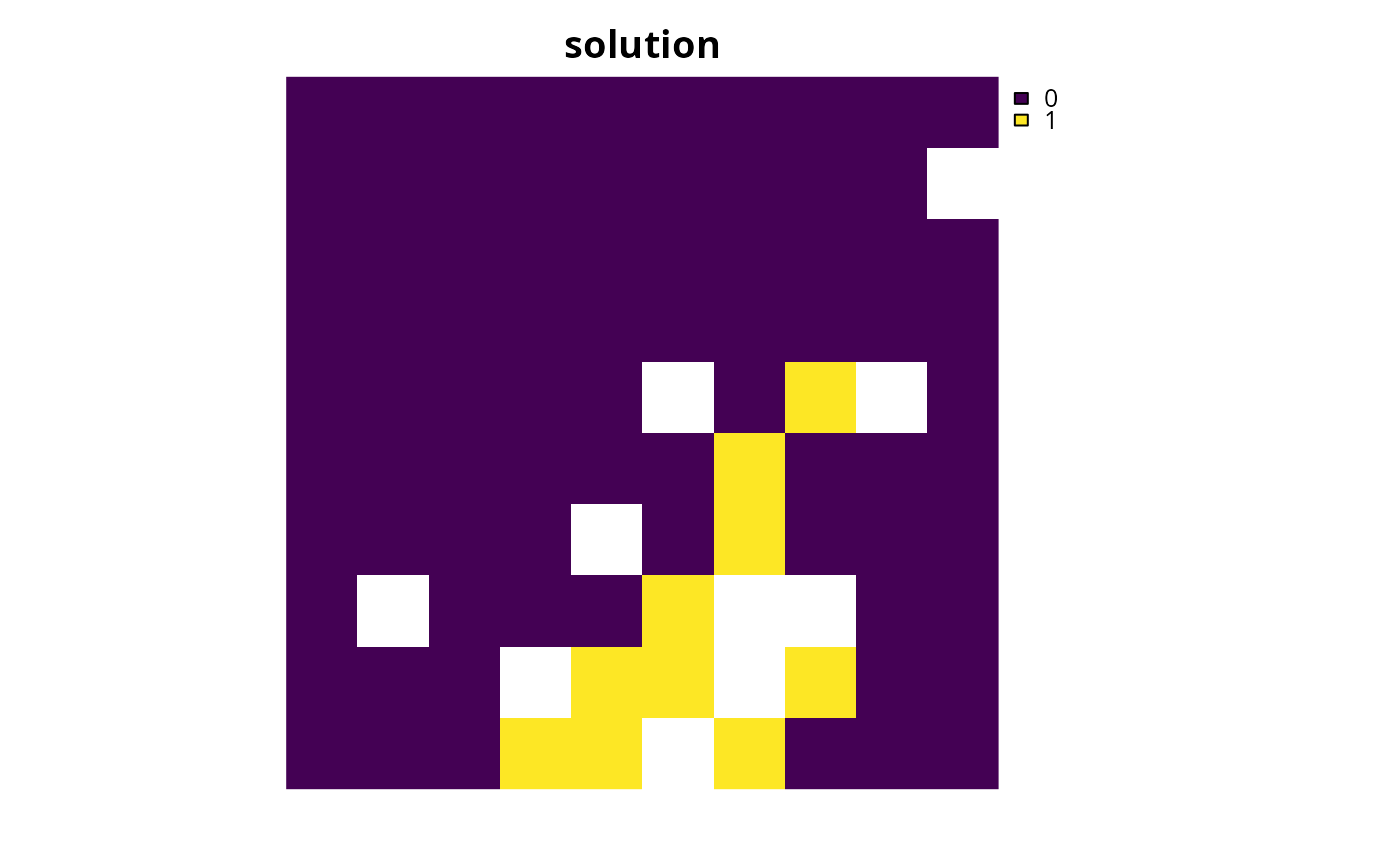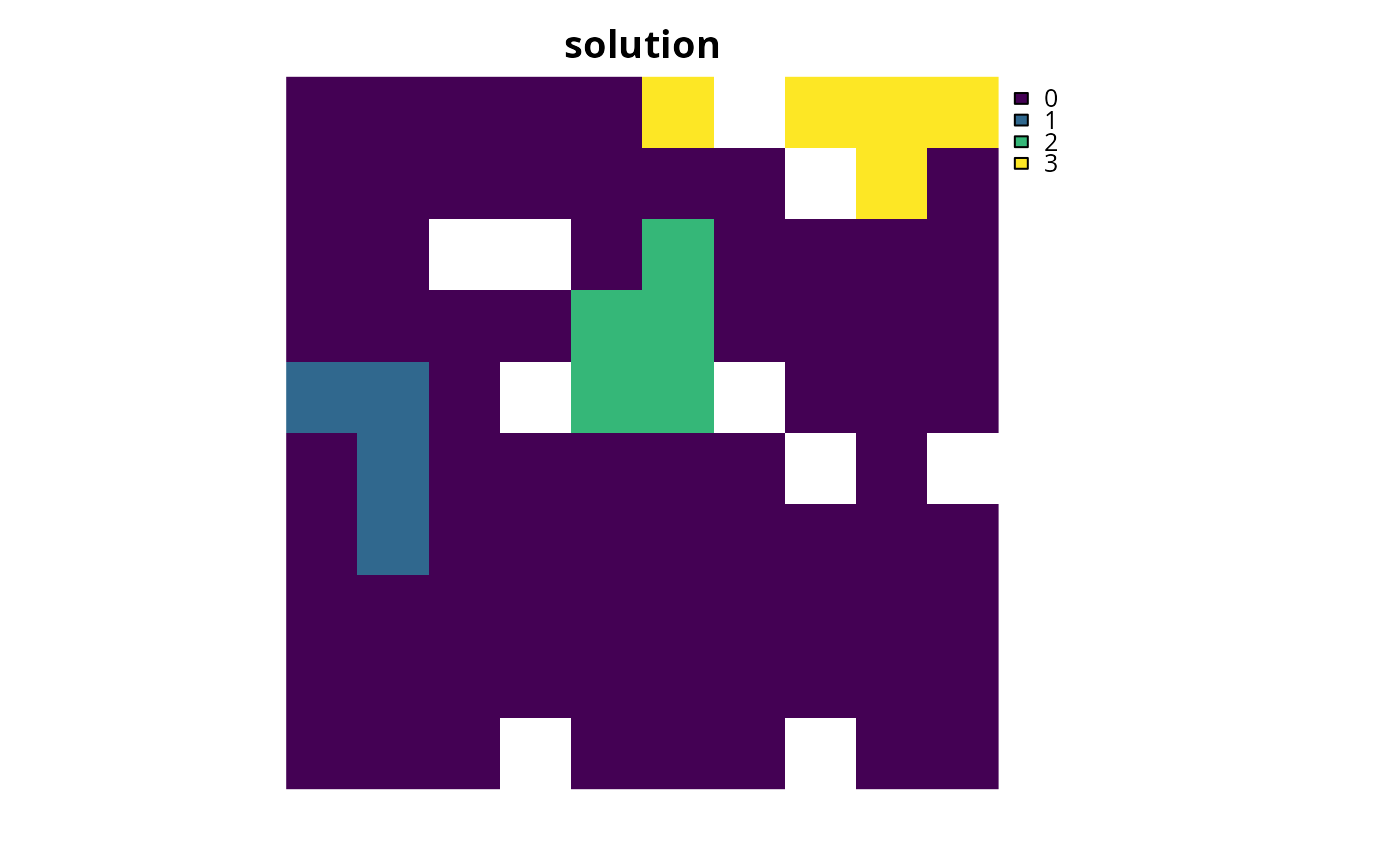Set the objective of a conservation planning problem() to minimize the cost of the solution whilst ensuring that all targets are met. This objective is similar to that used in Marxan and is detailed in Rodrigues et al. (2000).

add_min_set_objective(x)

## Arguments

x

problem() (i.e., ConservationProblem) object.

## Value

Object (i.e., ConservationProblem) with the objective added to it.

## Details

The minimum set objective -- in the the context of systematic reserve design -- seeks to find the set of planning units that minimizes the overall cost of a reserve network, while meeting a set of representation targets for the conservation features. This objective is equivalent to a simplified Marxan reserve design problem with the Boundary Length Modifier (BLM) set to zero. The difference between this objective and the Marxan software is that the targets for the features will always be met (and as such it does not use Species Penalty Factors).

## Mathematical formulation

This objective can be expressed mathematically for a set of planning units ($$I$$ indexed by $$i$$) and a set of features ($$J$$ indexed by $$j$$) as:

$$\mathit{Minimize} \space \sum_{i = 1}^{I} x_i c_i \\ \mathit{subject \space to} \\ \sum_{i = 1}^{I} x_i r_{ij} \geq T_j \space \forall \space j \in J$$

Here, $$x_i$$ is the decisions variable (e.g., specifying whether planning unit $$i$$ has been selected (1) or not (0)), $$c_i$$ is the cost of planning unit $$i$$, $$r_{ij}$$ is the amount of feature $$j$$ in planning unit $$i$$, and $$T_j$$ is the target for feature $$j$$. The first term is the objective function and the second is the set of constraints. In words this says find the set of planning units that meets all the representation targets while minimizing the overall cost.

See objectives for an overview of all functions for adding objectives. Also see targets for an overview of all functions for adding targets.

Other objectives: add_max_cover_objective(), add_max_features_objective(), add_max_phylo_div_objective(), add_max_phylo_end_objective(), add_max_utility_objective(), add_min_largest_shortfall_objective(), add_min_shortfall_objective()

## Examples

# \dontrun{
# set seed for reproducibility
set.seed(500)

data(sim_pu_raster, sim_features, sim_pu_zones_stack, sim_features_zones)

# create minimal problem with minimum set objective
p1 <- problem(sim_pu_raster, sim_features) %>%

# solve problem
s1 <- solve(p1)

# plot solution
plot(s1, main = "solution", axes = FALSE, box = FALSE)# create multi-zone problem with minimum set objective
targets_matrix <- matrix(rpois(15, 1), nrow = 5, ncol = 3)

p2 <- problem(sim_pu_zones_stack, sim_features_zones) %>%